Get instant live expert help with Excel or Google Sheets“My Excelchat expert helped me in less than 20 minutes, saving me what would have been 5 hours of work!”

#### Post your problem and you’ll get Expert help in seconds.

Your message must be at least 40 characters
Our professional Expert are available now. Your privacy is guaranteed.

# How to Highlight Duplicate Columns in Excel using a Formula

Working on a large database can often create confusions. It is not uncommon to create duplicate columns by mistake. Excel offers a great fix to diagnose if there are identical columns. We can do this by highlighting duplicate columns. We use the SUMPRODUCT function along with conditional formatting to do this. In this lesson, we will learn how to highlight duplicate columns in Excel.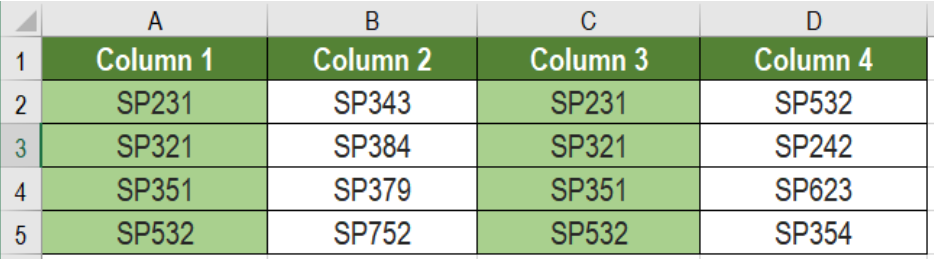Figure 1. Example of How to Highlight Duplicate Columns in Excel

## Formula

`=SUMPRODUCT ((row1=reference1) * (row2=reference) * (row3=reference3)) >1`

This formula is based on the SUMPRODUCT function. It counts how many times each value occurs in the table, in a row wise manner. The function will provide TRUE if the values are same in all the rows.

We use absolute row references. But the cell references are mixed, where the row is locked. This checks all instances of the row for duplicates.

## Setting up Data

The following database contains some sample text. Column A to D has these texts.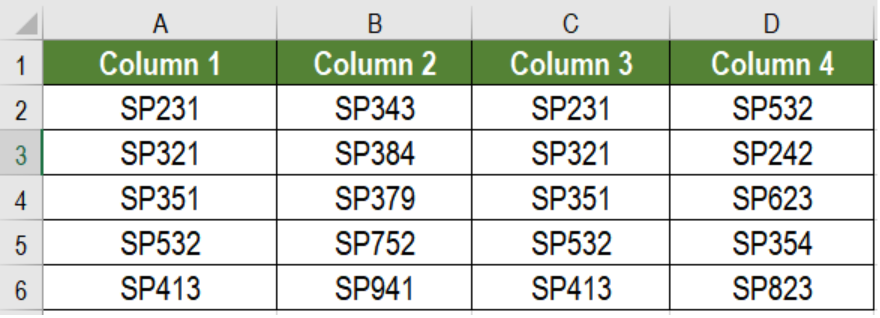Figure 2. The Data Set

To highlight duplicate columns, we need to:

• Click on cell A2. Drag the selection from cells A2 to D6.
• In the home tab of the ribbon, click Conditional Formatting > New Rule.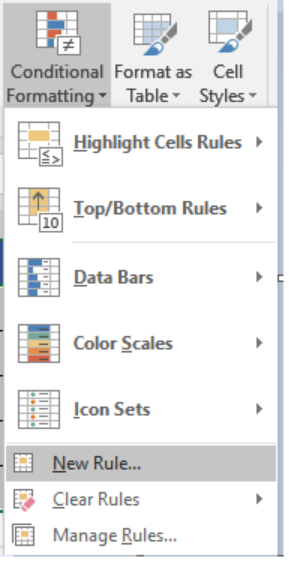Figure 3. Example of how to Apply Conditional Formatting

• Select Use a formula to determine which cells to format.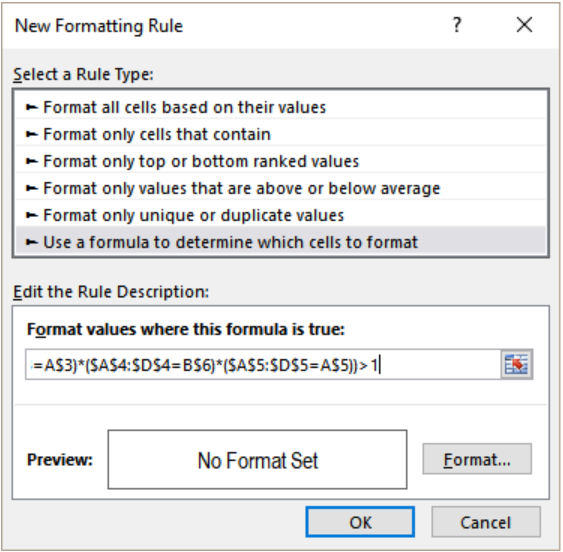Figure 4. Applying the Formula

• Next, we need to assign the formula` =SUMPRODUCT((\$A\$2:\$D\$2=A\$2)*(\$A\$3:\$D\$3=A\$3)*(\$A\$4:\$D\$4=A\$4)*(\$A\$5:\$D\$5=A\$5))>1`. This needs to be assigned in the Format values where this formula is true box,
• Next, we need to click on Format.
• Select Fill>Background Color and set it to the color of your choice.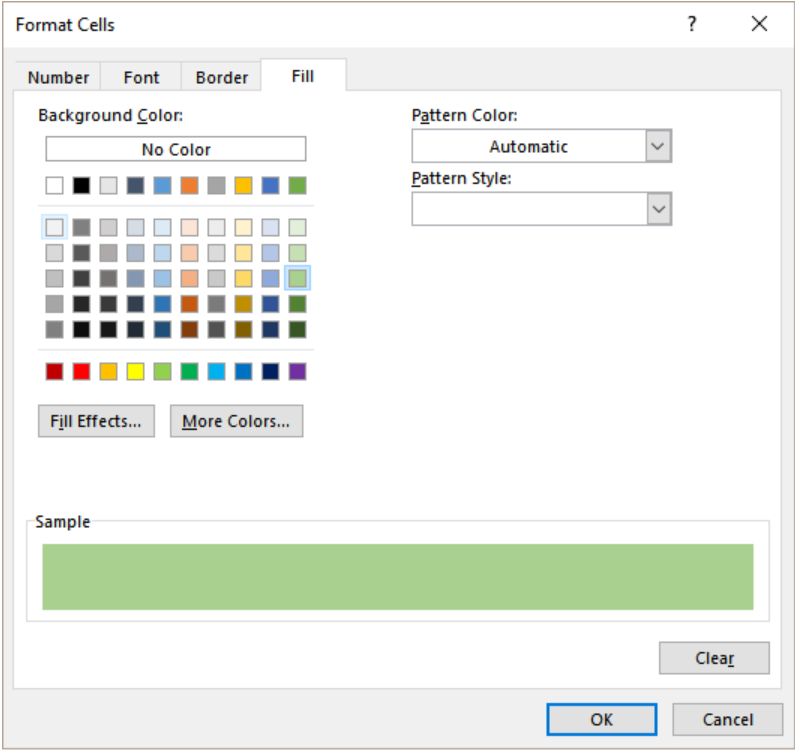Figure 5. Formatting the Display

• Click Ok twice.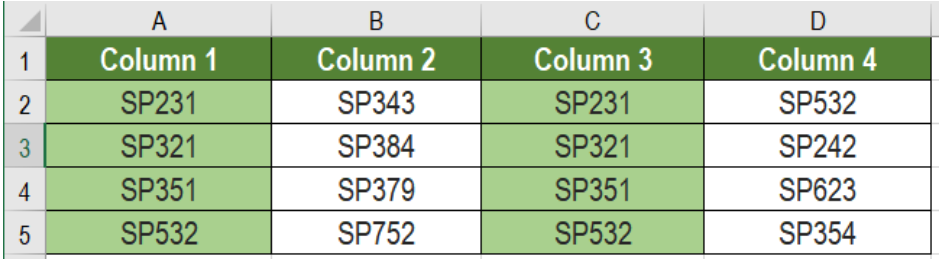Figure 6. The Final Result

This will highlight the duplicate columns B and C using the conditional formatting.

Most of the time, the problem you will need to solve will be more complex than a simple application of a formula or function. If you want to save hours of research and frustration, try our live Excelchat service! Our Excel Experts are available 24/7 to answer any Excel question you may have. We guarantee a connection within 30 seconds and a customized solution within 20 minutes.

### Did this post not answer your question? Get a solution from connecting with the expert.Another blog reader asked this question today on Excelchat:
Solution examplesI want to be able to alter the appearance (font color and fill) of a column of numbers using conditional formatting, based on being greater than or less than the numbers from another column. I am using Excel 2016. I am unable to get any formula to work. Please help!
Solved by I. J. in 59 minsI need assistance with an excel formula. I have a 12 month sheet that has a summary page on the 13 page. the monthly totals changes so on the summary page I want to sum the difference - eg. if Jan total is 1500, feb total is 1000, mar total is 800, I want the summary page to show the current amount of 800 when you place the formula to capture all months---showing the diminishing value rather than the sum of all the pages
Solved by E. L. in 60 minsI need a range of numbers to become the number in the next column
Solved by D. F. in 47 minsUse the Vlookup Function to complete the "employee" column of table 2. Use "job Id" from table 2 as your lookup_value(s) and table 1 as your reference.
Solved by C. H. in 16 minsI need to count number of dates from a timestamped date in column from the completed date column to show the number of days. I have not successfully been able to do this. I am a novice Excel user. Can you help?
Solved by O. B. in 15 mins## Subscribe to Excelchat.coAnother blog reader asked this question today on Excelchat: